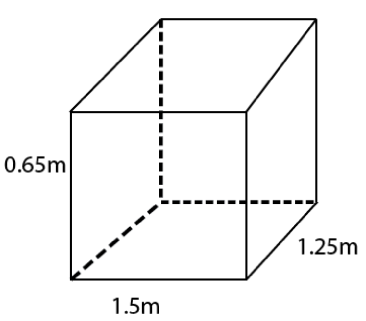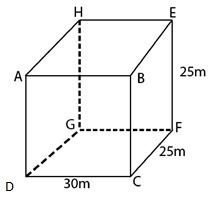# NCERT Solutions For Class 9 Math Chapter – 13 Exercise – 13.1

NCERT Solutions For Class 9 Math Chapter – 13 Exercise – 13.1

#### Experts at Sunstarup  have created the NCERT Solutions after extensive search on each topic Students can refer to this study study material to boost their confidence and attempt the second term exam smartly. The concepts are explained with steps, shortcuts to remember formula , tips and tricks to solve the numerical problems wisely and quickly.

Q1.

A plastic box 1.5 m long , 1.25 m wide and 65 cm and deep to be made. It is opened at the top. Ignoring th thickness of the plastic sheet , determine

(i) The area of the sheet required for making the box.

(ii) The cost of sheet for it, if a sheet measuring 1 m2 costs Rs 20.

Solution:-

(i) Here  length (l) = 1.5 m breadth (b) = 1.25 m

And height (h) = 65 cm = 65/100 m = 0.65 mIt is opened from the top.

It is surface area

[Lateral surface area] + [Base area]

= [2(l+h)h] + [lb]

= [2(1.50+1.25)0.65] m2 + [1.875] m2

= 5.45 m2

Area of the sheet required for making the box = 5.45 m2.

(ii) Cost of 1 m2 sheet = Rs 20.

Cost of 5.45 m2 sheet = Rs (20×5.45)

= Rs 109

Hence , cost of the required sheet = Rs 109

Q2. The length , breadth and height of a room are 5 m , 4m and 3 m respectively. Find the cost of white washing the walls of the room and the ceiling at the rate of Rs 17.50 per m2.

Solution:-

Length = 5 m

Height = 3 m

Area for white washing

[Lateral surface area] + [Area of the ceiling]

= [2(l+b)h] + [lxb]

= [2(5+4)x3] m2 + [5×4] m2

= 54 m2 + 20 m2

= 74 m2

Cost of white washing for 1 m2 area = Rs 7.50

Cost of white washing for 74 m2 area = Rs (7.50 x 74) = Rs 555

Thus, the required cost of white washing = Rs 555

Q3. The floor of a rectangular  hall has a perimeter 250 m. If the cost of painting the four walls at the rate of Rs 10 per m2 is Rs 15000 find the height of the hall.

Solution:-

Perimeter of the floor = 2(l+b)

= 2(l+b) = 250 m

Area of four walls = Lateral surface area = 2(l+b) x h = 250 h m2

Cost of painting the four walls = Rs (10x250h) = Rs 2500 h

2500h = 15000 = h = 15000/2500

H = 6 m

Q4. The paint in a certain container is sufficient to paint an area equal to 9.375 m2 How many bricks of dimensions 22.5 cm x 10 cm x 7.5 cm can be painted out of this container.

Solution:-

Total surface area of brick = 2[lb + bh + hl]

= 2[225×1(0) + (10×7.5) + )7.5 x 22.5)]

= 937.5 cm2

= 937.5/10000 m2.

Total surface area of n bricks = n x 937.5/100000 m2.

= n x 937.5/10000 m2.

N = 100

Thus , the required number of bricks = 100

Q5. A cubical box has each edge 10 cm and another cuboidal box is 12.5 cm long 10 cm wide and 8 cm high.

(i) Which box has the greater lateral surface area and by how much?

(ii) Which box has the smaller total surface area and by how much ?

Solution:-

Lateral surface area = 4a2.

= 4 x 102.

= 400 cm2.

Total surface area = 6a2

= 6 x 102 cm2

= 600 cm2

Lateral surface area = 2[l+b)xh]

= 2[12.5+10]x8

= 360 cm2.

Total surface area = 2[lb + bh + hl]

= 2[12.5×10 + 10 x 8 + 8x 12.5]

= 610 cm2.

(i) A cubical box has the grater lateral surface by (400-360) cm2 = 40 cm2.

(ii) Total surface area of a cubical box is smaller than the cuboidal box by (610-600) cm2

= 10 cm2.Q6. A small indoor greenhouse (herbarium) is made entirely of glass panes held together with tape It is 30 cm  long 25 cm high

(i)  What is the area of the glass ?

(ii) How much  of tape is needed for all the 12 edges ?

Solution:-(i) Surface area of the herbarium (glass)

= 2[lb + bh + hl]

= 2[30×25 + 25 x 25 + 25 x 30 ] cm2.

= 2 cm2.

= 4250 cm2.

Thus the required area of the glass = 4250 cm2.

(ii) Total length of 12 edges = 4l + 4b + 4h

= 4(l + b + h)

= 4(30+25+25)

= 4 x 80 cm

= 320 cm

Thus , the required length of tape = 320 cm

Q7. Shanti Sweets Stall was placing an order for making carboard boxes for packing their sweets. Two sizes of boxes were required The bigger of dimensions 25 cm x 20 cm x 5 cm and the smaller of dimensions 15 cm x 12 cm x 5 cm

For all the overlaps 5% of the total surface area is required extra. If the cost of the cardboard is Rs 4 for 1000 cm2 find the cost of cardboard required for supplying 250 boxes of each kind.

Solution:-

Total surface area of a box = 2(lb + bh + hl)

= 2[25×20 + 20×5 + 5×25] cm2.

= 2[500 + 100 + 125] cm2.

= 1450 cm2.

Total surface are of 250 boxes = (250×1450) cm2.

= 362500 cm2.

For smaller box:

Total surface area of a box = 2[lb + bh + hl]

= 2[15×12 + 12×5 + 5×15]

= 630 cm2.

Total surface area of 250 boxes = (250×630) cm2

= 157500 cm2 .

Total surface area of both type of boxes = 362500 + 157500

= 520000 cm2.

Area of overlaps = 5% of [Total surface area of 250 boxes of each type] + [Area for overlaps]

= 520000 cm2 + 26000 cm2

= 546000 cm2.

Cost of 1000 cm2 cardboard = Rs 4.

Cost of 546000 cm2 cardboard = Rs 4 x 546000/1000

Rs = 2184

Q8. Praveen wanted to make a temporary shelter, for her car, by making a box – like structure with tarpaulin that covers all the the four sides and the top of the car Assuming that the stitching margins are very small and therefore negligible how much tarpaulin would be required to make the shelter of height 2.5 m with base dimensions 4 m x 3 m?

Solution:-

The surface area of the cuboid , excluding the base

= [Lateral surface area] + [Area of ceiling]

=[2(l+b)h] + [lb]

= [2(4+3)x2.5] m2 + [4×3] m2

= 47 m2.

Thus , 47 m2 tarpaulin would be required.